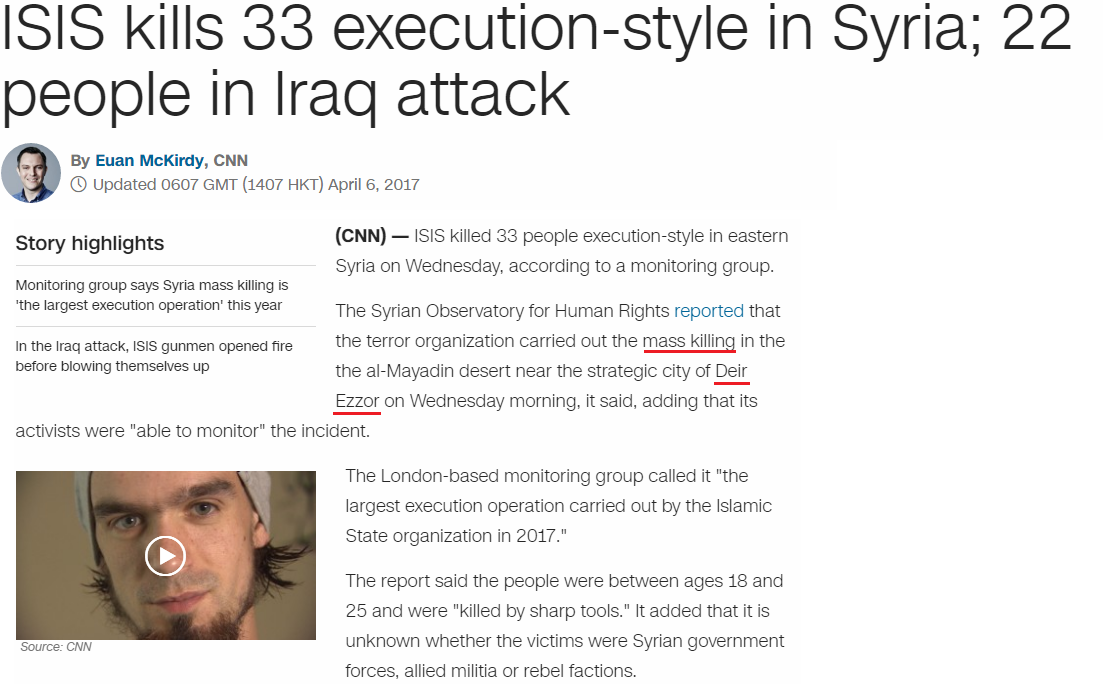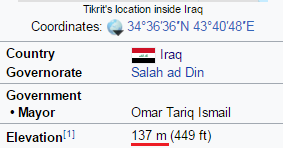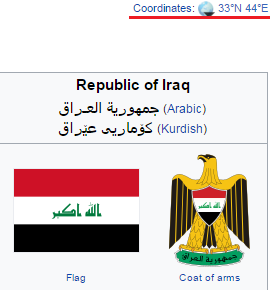CipherApr 6, 2017

CNN - ISIS kills 33 in Syria and 22 in Iraq (not really)...

http://edition.cnn.com/2017/04/06/middleeast/isis-deir-ezzor-killings/index.htmlThis is coming on 4/6/2017 = 4+6+2+1+7 = 20 - "ISIS" = 9+1+9+1 = 20 (Full Reduction)
4/6/17 - 4+6+17 = 27 - Iraq = 9+9+1+8 = 27 (Full Reduction), Syria = 1+7+9+9+1 = 27 (Full Reduction)

This story is split in two parts so we have to start with Syria
ISIS kills 33 execution-style in Syria = 9+1+9+1 + 2+9+3+3+1 + 5+6+5+3+3+2+9+6+5 + 1+2+7+3+5 + 9+5 + 1+7+9+9+1 = 141 (Full Reduction)
Thirty three = 7+19+18+9+7+2 + 7+19+9+22+22 = 141 (Reverse Ordinal)
ISIS "killed 33 people in Deir ez-Zor in Syria... here's why they chose this placeDeir Ezzor = 5+4+9+9 + 4+1+1+3+9 = 45 (Reverse Reduction)
mass killing = 4+1+1+1 + 2+9+3+3+9+5+7 = 45 (Full Reduction)

Iraq = 9+18+1+17 =
This supposedly took place in Iraqi city of "Tikrit" = 2+9+2+9+9+2 = 33 (Full Reduction)
"Iraq killings" = 9+9+1+8 + 2+9+3+3+9+5+7+1 = 66 (Full Reduction)
"Thirty three" = 2+8+9+9+2+7 + 2+8+9+5+5 = 66 (Full Reduction)Notice the elevation of 137 meters (33rd prime number is 137) and in this article they point out Tikrit is the "birthplace" of "Saddam Hussein" = 19+1+4+4+1+13 + 8+21+19+19+5+9+14 = 137 (English Ordinal)
This is also coming on a date with 47 numerology (4+6+20+17) - "Saddam Hussein" = 1+1+4+4+1+4 + 8+3+1+1+5+9+5 = 47 (Full Reduction)
I find it very interesting how they are saying that there are 22 people killed in Iraq and this is coming 22 days before birthday of Saddam Hussein...this might be the reason they are saying 22 people got killed today, these people are very insane and tributes like this are very commonThey give us very interesting coordinates for the republic of Iraq on WikipediaThis story is coming on "Wednesday" = 4+4+5+4+4+8+5+8+2 = 44 (Reverse Reduction)